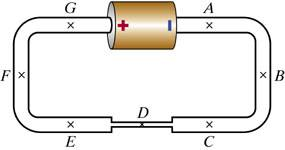# Electric Field of Single-Battery Circuit with Thin/Thick Wires

## Homework StatementThe circuit shown above consists of a single battery, whose emf is 1.4 V, and three wires made of the same material, but having different cross-sectional areas. Each thick wire has cross-sectional area 1.4e-6 m2, and is 21 cm long. The thin wire has cross-sectional area 6.4e-8 m2, and is 7.5 cm long. In this metal, the electron mobility is 6e-4 (m/s)/(V/m), and there are 8e+28 mobile electrons/m3.

Use the appropriate equation(s), plus the equation relating electron current to electric field, to solve for the factor that goes in the blank below:
EF = ? * ED

Use the appropriate equation(s) to calculate the magnitude of ED
ED = ?

Use the appropriate equation(s) to calculate the electron current at location D in the steady state

???

## The Attempt at a Solution

Already tried it, and I can't do it. E = emf/L doesn't work here.

I've looked everywhere to try and find equations that can help me solve it, or at least relate the thin and thick wire. My physics book seems to skip this whole section (I'm using a different physics book than my class, which kind of sux for me, but I didn't want to buy another one).

Last edited by a moderator:

I solved it all on my own. I'll post the solution here so people will know how to do it if they come across it.

i = naME
i = electron current
n = electron density
a = area
M = electron mobility
E = electric field
For the thin and thick wire, the electron current for both of them is constant. So you can put the two together.
nA(thick)ME(thick) = nA(thin)ME(thin)
A(thick)E(thick) = A(thin)E(thin), n and M are constants, too.
E(thick) = [A(thin)/A(thick)]E(thin)
A(thin) = 6.4E-8 m^2
A(thick) = 1.4E-6 m^2
E(thick) = 0.046E(thin)

You have the equation:
0 = 1.4 - EF*0.21 - ED*0.075 - EF*0.21 [Found by applying the Loop Rule]
E(thick) = 0.046E(thin).
So, EF = E(thick) and ED = E(thin) from the diagram. Plug it in.
0 = 1.4 - 2(0.21)(0.046)E(thin)
E(thin) = 14.8 V/M

You have i=naME to find i. So plug that in.
M = 6E-4
n = 8E28
A(thin) = 6.4E-8
E = 14.8
i = (6E-4)(8E28)(6.4E-8)(14.8) = 4.5E19 electrons / second

0 = 1.4 - 2(0.21)(0.046)E(thin)
E(thin) = 14.8 V/M

If you solve that you don't get 14.8, your def missing something here!